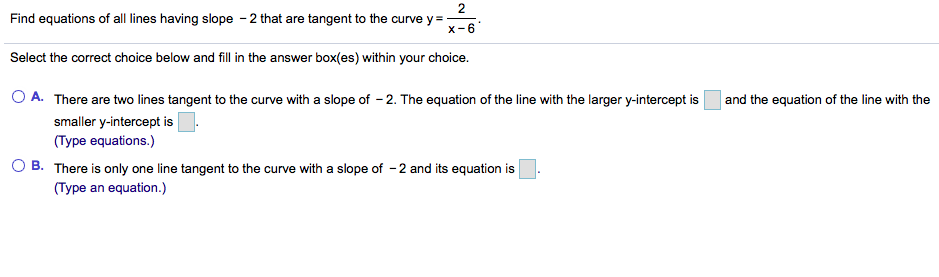# 2 Find equations of all lines having slope 2 that are tangent to the curve y - x-6 Select the correct choice below and fill in the answer box(es) within your choice. A. There are two lines tangent to the curve with a slope of 2. The equation of the line with the larger y-intercept is and the equation of the line with the smaller y-intercept is (Type equations.) O B. There is only one line tangent to the curve with a slope of -2 and its equation is (Type an equation.)

Question

can you help me step by step pleasehelp_outlineImage Transcriptionclose2 Find equations of all lines having slope 2 that are tangent to the curve y - x-6 Select the correct choice below and fill in the answer box(es) within your choice. A. There are two lines tangent to the curve with a slope of 2. The equation of the line with the larger y-intercept is and the equation of the line with the smaller y-intercept is (Type equations.) O B. There is only one line tangent to the curve with a slope of -2 and its equation is (Type an equation.) fullscreen

## Expert Answer

### Want to see this answer and more?

Experts are waiting 24/7 to provide step-by-step solutions in as fast as 30 minutes!*

*Response times may vary by subject and question complexity. Median response time is 34 minutes for paid subscribers and may be longer for promotional offers.
Tagged in
Math
Calculus

### Derivative© 2021 bartleby. All Rights Reserved.